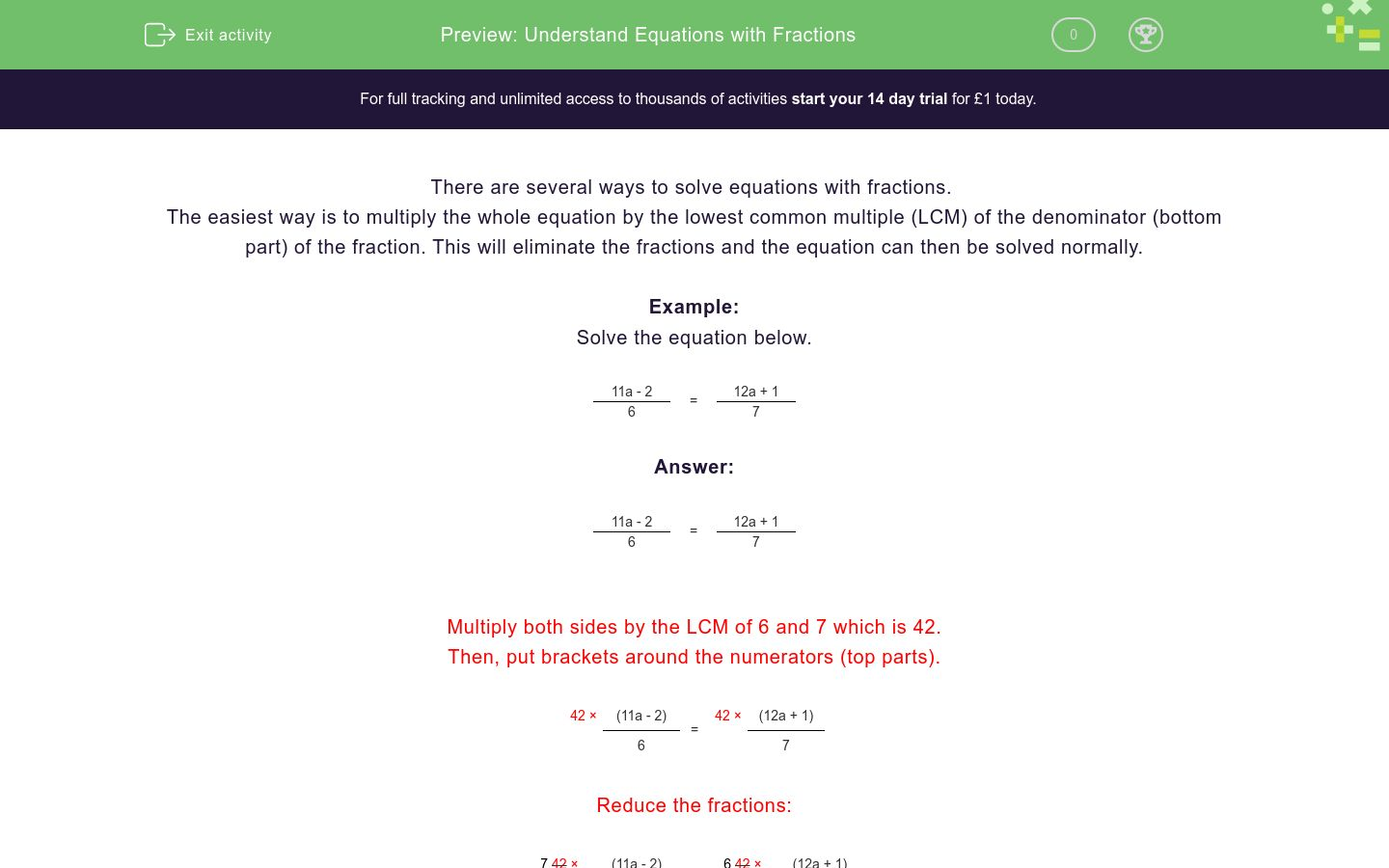# Equations with Fractions (3)

In this worksheet, students solve equations with fractions.Key stage:  KS 4

Curriculum topic:  Algebra

Difficulty level:### QUESTION 1 of 10

There are several ways to solve equations with fractions.

The easiest way is to multiply the whole equation by the lowest common multiple (lcm) of the denominators (bottom parts) of the fraction.  This will eliminate the fractions and the equation can then be solved normally.

Example

Solve the following equation for a:

 11a - 2 = 12a + 1 6 7

Answer

 11a - 2 = 12a + 1 6 7

Multiply both sides by the lcm of 6 and 7 which is 42.

Put brackets around the numerators (top parts).

 42 × (11a - 2) = 42 × (12a + 1) 6 7

Reduce the fractions

 7 42 × (11a - 2) = 6 42 × (12a + 1) 6 7

Simplify

7 (11a - 2) = 6 (12a + 1)

Multiply out the brackets

77a - 14 = 72a + 6

Add 14 to both sides

77a - 14 + 14 = 72a + 6 + 14

Simplify

77a = 72a + 20

Subtract 72a from both sides

77a -72a = 72a + 20 - 72a

Simplify

5a = 20

Divide both sides by 5

5a ÷ 5 = 20 ÷ 5

Simplify

a = 4

Solve the following equation for a:

 a + 2 = a + 4 5 7

(just write the value of a)

Solve the following equation for a:

 a + 2 = a + 12 4 9

(just write the value of a)

Solve the following equation for a:

 a + 1 = a + 6 7 12

(just write the value of a)

Solve the following equation for a:

 a - 1 = a + 4 3 8

(just write the value of a)

Solve the following equation for a:

 a - 4 = a - 1 8 11

(just write the value of a)

Solve the following equation for a:

 a - 4 = a - 11 3 10

(just write the value of a)

Solve the following equation for a:

 3a + 2 = 6a + 11 2 5

(just write the value of a)

Solve the following equation for a:

 4a + 3 = 6a + 7 3 5

(just write the value of a)

Solve the following equation for a:

 7a + 3 = 5a - 1 12 7

(just write the value of a)

Solve the following equation for a:

 7a - 10 = 8a - 5 5 7

(just write the value of a)

• Question 1

Solve the following equation for a:

 a + 2 = a + 4 5 7

(just write the value of a)

CORRECT ANSWER
3
• Question 2

Solve the following equation for a:

 a + 2 = a + 12 4 9

(just write the value of a)

CORRECT ANSWER
6
• Question 3

Solve the following equation for a:

 a + 1 = a + 6 7 12

(just write the value of a)

CORRECT ANSWER
6
• Question 4

Solve the following equation for a:

 a - 1 = a + 4 3 8

(just write the value of a)

CORRECT ANSWER
4
• Question 5

Solve the following equation for a:

 a - 4 = a - 1 8 11

(just write the value of a)

CORRECT ANSWER
12
• Question 6

Solve the following equation for a:

 a - 4 = a - 11 3 10

(just write the value of a)

CORRECT ANSWER
1
• Question 7

Solve the following equation for a:

 3a + 2 = 6a + 11 2 5

(just write the value of a)

CORRECT ANSWER
4
• Question 8

Solve the following equation for a:

 4a + 3 = 6a + 7 3 5

(just write the value of a)

CORRECT ANSWER
3
• Question 9

Solve the following equation for a:

 7a + 3 = 5a - 1 12 7

(just write the value of a)

CORRECT ANSWER
3
• Question 10

Solve the following equation for a:

 7a - 10 = 8a - 5 5 7

(just write the value of a)

CORRECT ANSWER
5
---- OR ----

Sign up for a £1 trial so you can track and measure your child's progress on this activity.

### What is EdPlace?

We're your National Curriculum aligned online education content provider helping each child succeed in English, maths and science from year 1 to GCSE. With an EdPlace account you’ll be able to track and measure progress, helping each child achieve their best. We build confidence and attainment by personalising each child’s learning at a level that suits them.

Start your £1 trial